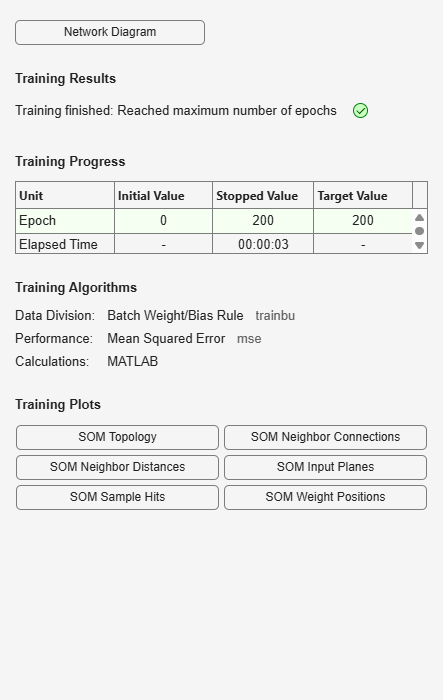# plotsomplanes

Plot self-organizing map weight planes

## Syntax

```plotsomplanes(net) ```

## Description

`plotsomplanes(net)` generates a set of subplots. Each `i`th subplot shows the weights from the `i`th input to the layer’s neurons, with the most negative connections shown as black, zero connections as red, and the strongest positive connections as yellow.

The plot is only shown for layers organized in one or two dimensions.

This plot supports `SOM` networks with `hextop` and `gridtop` topologies, but not `tritop` or `randtop`.

This function can also be called with standardized plotting function arguments used by the function `train`.

## Examples

### Plot SOM Weight Planes

```x = iris_dataset; net = selforgmap([5 5]); net = train(net,x); plotsomplanes(net)```